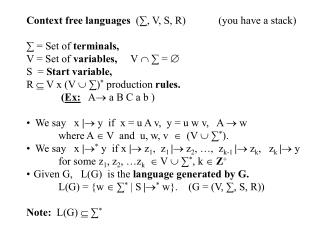DownloadDownload PresentationContext free languages (∑, V, S, R) (you have a stack) ∑ = Set of terminals, V = Set of variables, V  ∑ =

# Context free languages (∑, V, S, R) (you have a stack) ∑ = Set of terminals, V = Set of variables, V  ∑ =

Télécharger la présentation## Context free languages (∑, V, S, R) (you have a stack) ∑ = Set of terminals, V = Set of variables, V  ∑ =

- - - - - - - - - - - - - - - - - - - - - - - - - - - E N D - - - - - - - - - - - - - - - - - - - - - - - - - - -
##### Presentation Transcript

1. Context free languages (∑, V, S, R) (you have a stack) • ∑ = Set of terminals, • V = Set of variables, V  ∑ =  • S = Start variable, • R  V x (V  ∑)* production rules. • (Ex: A a B C a b ) • We say x | y if x = u A v, y = u w v, A  w where A  V and u, w, v  (V  ∑*). • We say x |* y if x | z1, z1 | z2, …, zk-1 | zk, zk | y • for some z1, z2, …zk  V  ∑*, k  Z+ • Given G, L(G) is the language generated by G. • L(G) = {w  ∑* | S|* w}. (G = (V, ∑, S, R)) • Note: L(G)  ∑*

2. S A B a A b B . . . . . . . . . . . . If we have rules: SA B, A aA, A , B bB, B , We might have:S | AB | aAB | aAbB | … Which we represent as: (because order doesn't matter) Notice this language is a* b* .

3. Context Free Grammar (CFG) • G = (V, ∑, R, S) • V = Variables, ∑ = Terminals, R = Production Rules, • Where S = Start State, S  V, and R  V x (V  ∑)* • L = {an bn | n  N  {0}} is not regular. Is it context-free? Yes. The grammar G = (V, ∑, R, S), where • ∑ = {a, b}, V = {S}, R = { S | a S b | } generates L ! • L = {an bm | n  m}: R = {S | a S b | a S | }. • L = {an bm | n > m}: R = {S | a A ; A | a A b |  | a A}.

4. Thm: Every regular language is context free. • Pf: Let L be regular. Then  a finite automaton • (Q, ∑, , q0, F). We will build a CFG for L. • The grammar is ( V, ∑1, R, S1), • where V = Q  S1, ∑1 = ∑, S1 = Sq0, • and if Q = {q0, q1, …}, V = {Sq0, Sq1, …}. • Finally, for every transition (qi, ) = qj , • write Sqi  Sqj as a rule. Finally, add the rule • Sqk  if qk  F. • Now we need to argue that this grammar accepts L. • Why is this the case?

5. Thm: The union of two context free languages is also context free. Pf: Let G1 = (V1, ∑1, R1, S1) and G2 = (V2, ∑2, R2, S2) be CFG's. We want to create G = (V, ∑, R, S) s.t. L (G) = L(G1)  L (G2). where the rules are: R = {S S1 | S2}  R1  R2 , ∑ = ∑1 ∑2, and V = S  V1  V2 . x

6. S S1 S2 L1L2 • Thm: Concatenation of context-free languages is context free. • Pf: Given G1 = (V1, ∑1, R1, S1) and G2 = (V2, ∑2, R2, S2), CFGs, define a new grammar G = (V, ∑, R, S): • R = {SS1 S2}  R1  R2, • ∑ = ∑1 ∑2 , V = V1  V2  S. x • Thm: CFG's closed under * • Pf: Given G=(V, S, R, S), define a new grammar • G = (V, ∑, R1, S) where • R1 = {S S S | }  R. x

7. Note: • L = { w wR | w  ∑*} is context free since: S a S a | b S b |  generates it. • L = { w w | w  ∑*} is not context free. • L = { an bn cn | n Z+} is not context free. • (we’ll prove these next time!) • Careful: • L3 = {an bn cm | n, m N  {0}} is context free: • S a S1 b S2 |  | S2S2 c S2 |  (*or use concatenation!)S1 a S1 b |  • So is L4 = {am bn cn | n, m N  {0}}. • ButL5 = L3 L4 = { an bn cn | N  {0}}, so this shows CFG's • aren't closed under intersection. • (And thus they aren’t closed under complement either.)